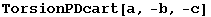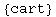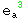8. Charts

Thus far, everything is a field on a manifold or a function of a parameter. We shall want to express scalar fields as functions of coordinate fields. To do this we need a chart

DefChart            Define a basis
\$Bases
List of currently defined bases
ChartQ                Check existence of a given basis name

Definition of a charts.

This whole section has not been implemented yet. At the moment, there is no easy way to restrict fields to points, for example. DefChart, however, does work. We need to supply a list of scalars (the coordinates)

In:=Notice that now the torsion tensor vanishes and the Christoffel is symmetric.

In:=Out=In:=Out=In:=Out=In:=Out=In:=Out=In:=Out=Created by Mathematica  (May 16, 2008)